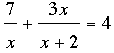SEARCH HOMEMath Central Quandaries & QueriesQuestion from John, a student: Solve each equation. Check each solution. 1/8+5x/x+2=5/2 10/2y+8 - 7y+8/y(squared)-16 = -8/2y-8Hi John,

Each of these problems require finding a common denominator, simplifying the expression and then solving the resulting equation. I'm going to illustrate withUsing the common denominator of x(x + 2) on the left side this simplifies to

7(x + 2) + x(3x) = 4x(x + 2)
-x2 - x + 14 = 0

Solving this using the general quadratic gives me

x = 1/2 (-1 ± √65)

Now try your problems,
HarleyMath Central is supported by the University of Regina and The Pacific Institute for the Mathematical Sciences.